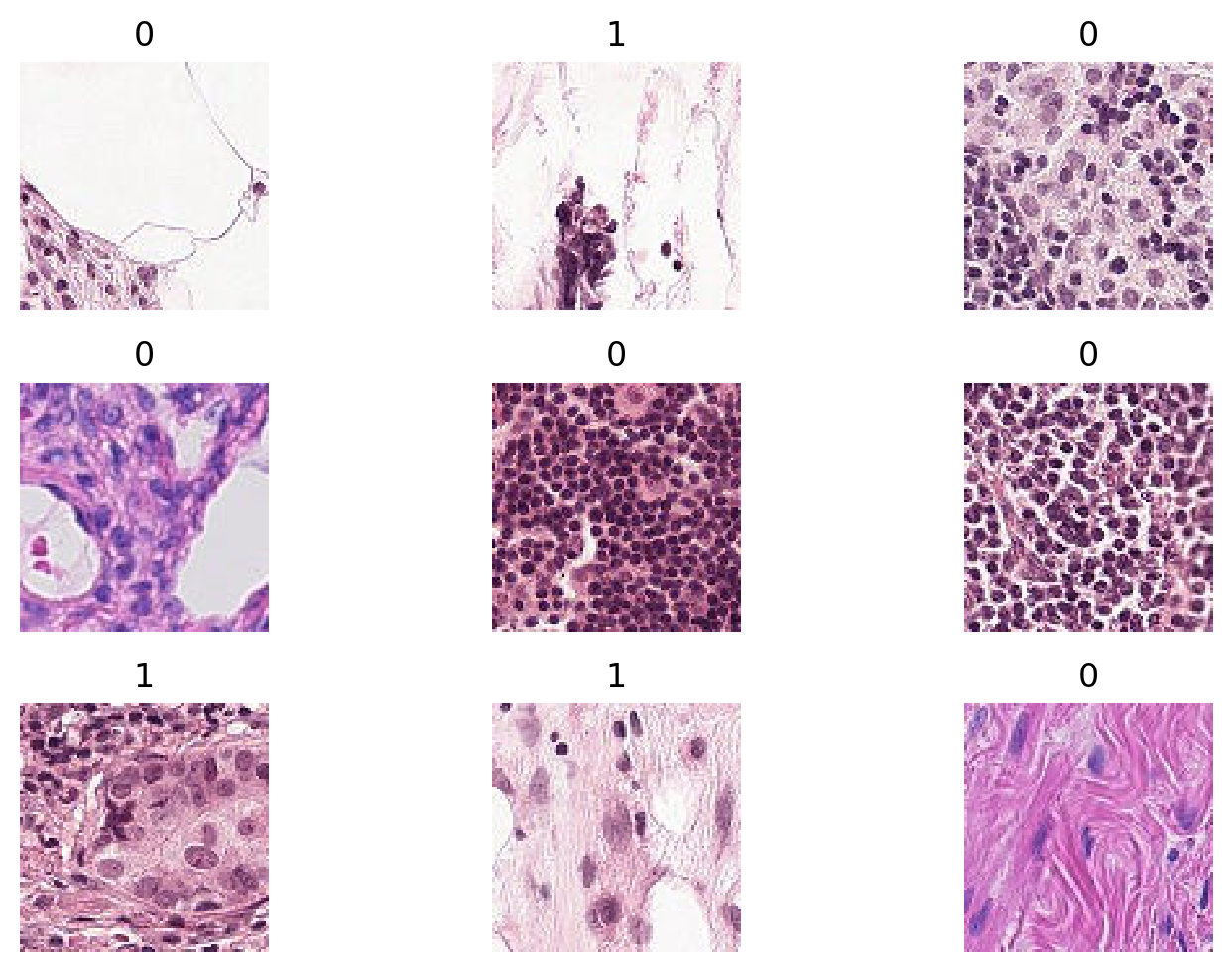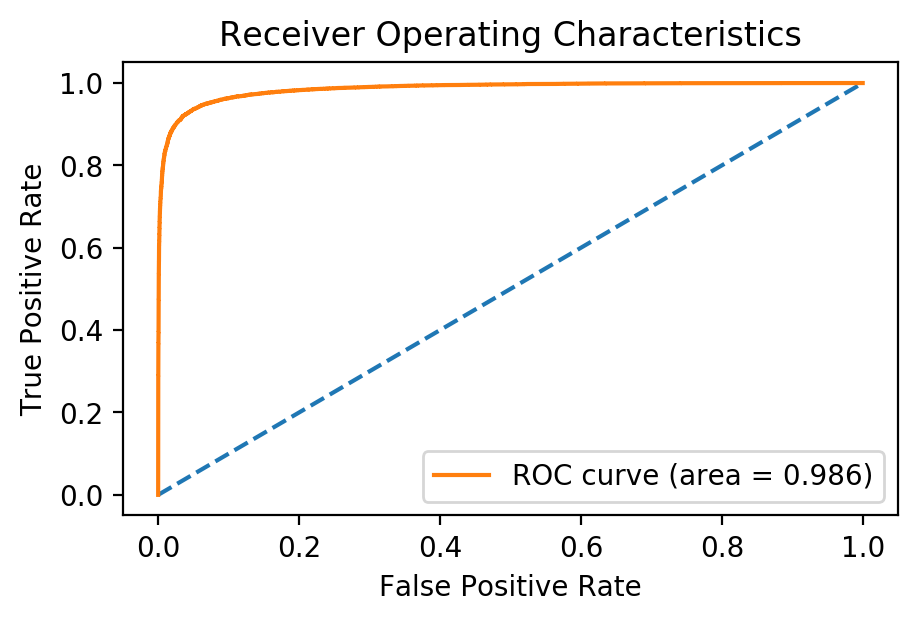In this post we will be using a method known as transfer learning in order to detect metastatic cancer in patches of images from digital pathology scans.

%matplotlib inline
import pandas as pd
import torch
import matplotlib.pyplot as plt
import cv2
import numpy as np
plt.rcParams["figure.figsize"] = (5, 3) # (w, h)
plt.rcParams["figure.dpi"] = 200


## Data

The data we will be using is located on Kaggle in the dataset Histopathologic Cancer Detection. There is a file train_labels.csv that contains all of the image names and classification labels. Let’s load the CSV file in and take a look at the first few images:

df = pd.read_csv("../data/train_labels.csv")
fig = plt.figure(figsize=(8,5))
for i in range(9):
fname = f"../data/train/{df.id[i]}.tif"
plt.title(df.label[i]); plt.axis("off")
fig.tight_layout()In this dataset, an image is marked 1 if the center 32x32px region contains at least one pixel of tumor tissue. Otherwise, it is marked 0. To the untrained eye, it is hard to notice any specific differences between the positive and negative images. Lucky for us, our computer will do all the hard work. Before we build our model, let’s create a PyTorch DataSet that will allow us easily feed our images and labels into the model for training.

from torch.utils.data import DataLoader, Dataset

class HistopathologicDataset(Dataset):
self.fnames = [f"{datadir}/{i}.tif" for i in df.id]
self.labels = df.label.tolist()
self.transform = transform

def __len__(self):
return len(self.fnames)

def __getitem__(self, index):
if self.transform:
img = self.transform(img)
return img, self.labels[index]


### Transforms

Before we load our datasets, we will need to create a series of transforms. Transforms are used to convert the image into a format that our model can understand. We first ensure the image is the appropriate size, and then convert it convert it to a Tensor, which is a multi-dimensional matrix that can be used on both CPUs and GPUs. For our training set, we will use a few additional transforms that randomly flip and rotate the image. These random transforms help increase the diversity of our training set, a technique known as Data Augmentation.

from torchvision import transforms, models

normalize = transforms.Normalize([0.485, 0.456, 0.406], [0.229, 0.224, 0.225])

train_transforms = transforms.Compose([
transforms.ToPILImage(),
transforms.RandomHorizontalFlip(),
transforms.RandomVerticalFlip(),
transforms.RandomRotation(20),
transforms.CenterCrop((49, 49)),
transforms.ToTensor(),
normalize,
])

valid_transforms = transforms.Compose([
transforms.ToPILImage(),
transforms.CenterCrop((49, 49)),
transforms.ToTensor(),
normalize,
])


Now we can load our datasets. We will load in 80% of the images as our training dataset, and use the other 20% to evaluate the model as it trains:

split = int(0.8 * len(df))
batch_size = 64
train_dataset = HistopathologicDataset(df[:split], "../data/train", train_transforms)
valid_dataset = HistopathologicDataset(df[split:], "../data/train", valid_transforms)


## Model

Now that we have our datasets, we can build our model!

### Transfer Learning

Visual object recognition has been an ongoing problem within the computer vision community. As a result, researchers compete to produce state-of-the-art results on datasets such as ImageNet which consists of millions of images in 1000 object categories. One type of neural network architecture commonly used in the challenge is a residual neural network (ResNet), and we can load a pre-trained version with just one line!

resnet = models.resnet50(pretrained=True)


Now we have a model that can accurately classify images of 1000 different objects, including everything from cats, to pencils, to airplanes. How does this help us with histopathologic tissue images? In the process of learning to distinguish between the different objects in ImageNet, the middle layers of the network learn to identify different features, such as lines, curves, and other patterns that help it make a prediction in the last layer. We can use these same features on our own dataset simply by replacing the last layer with a few layers of our own. This technique is known as transfer learning.

from torch import nn

in_features = resnet.fc.in_features
num_hidden = 512

nn.Flatten(),
nn.BatchNorm1d(in_features),
nn.Dropout(0.5),
nn.Linear(in_features=in_features, out_features=num_hidden),
nn.ReLU(),
nn.BatchNorm1d(num_hidden),
nn.Dropout(0.5),
nn.Linear(in_features=num_hidden, out_features=2),
)

model = nn.Sequential(
nn.Sequential(*list(resnet.children())[:-2]),
)


Now that we have created our model, we are ready for training.

## Training

First we’ll need to specify that we’d like to use our GPU for training if possible. This will speed up the the training process by many orders of magnitude:

device = torch.device('cuda:0' if torch.cuda.is_available() else 'cpu')


Now we’ll write a couple helper functions to train a batch of images, and compute the accuracy on the validation set:

def train(train_loader, model, criterion, optimizer, scheduler):
total_loss = 0.0
model.train()
for i, (images, labels) in enumerate(train_loader):
print(f"Training: {i}/{num_batches}", end="\r")

scheduler.step()
images = images.to(device)
labels = labels.to(device)
outputs = model(images) # forward pass
loss = criterion(outputs, labels)
total_loss += loss.item() * images.size(0)
loss.backward()  # backprogagation
optimizer.step()

model.eval()
total_correct = 0
total_loss = 0.0
for i, (images, labels) in enumerate(valid_loader):
print(f"Validation: {i}/{num_batches}", end="\r")

images = images.to(device)
labels = labels.to(device)
outputs = model(images)
loss = criterion(outputs, labels)
_, preds = torch.max(outputs, 1)
total_correct += torch.sum(preds == labels.data)
total_loss += loss.item() * images.size(0)



Now we’ll write our main training loop. For determining the learning rate of each training batch, we’ll use a technique known as the 1 cycle policy. First outlined in Leslie Smith’s A disciplined approach to neural network hyper-parameters, the 1 cycle policy consists of training in two steps, first going from a low to high learning rate, second going from high to low. The result of this approach is a significantly reduced training time. At the time of writing there is an open pull request to implement the policy in PyTorch, but for now I will copy the code to onecyclelr.py

from onecyclelr import OneCycleLR

criterion = nn.CrossEntropyLoss()
optimizer = torch.optim.SGD(model.parameters(), lr=1e-3, momentum=0.9)
scheduler = OneCycleLR(optimizer, max_lr=1e-2, train_dl=train_loader, num_epochs=num_epochs)
print("epoch\ttrain loss\tvalid loss\taccuracy")
for epoch in range(num_epochs):
train_loss = train(train_loader, model, criterion, optimizer, scheduler)
valid_loss, valid_acc = validate(valid_loader, model, criterion)
print(f"{epoch}\t{train_loss:.5f}\t\t{valid_loss:.5f}\t\t{valid_acc:.3f}")


Now let’s train for 20 epochs. We should be able to see the training loss, validation loss, and accuracy improve with each epoch:

model = model.to(device)

epoch	train loss	valid loss	accuracy
0	0.34798		0.27957		0.884
1	0.28280		0.26708		0.890
2	0.26309		0.22680		0.910
3	0.24658		0.21298		0.915
4	0.23179		0.21183		0.916
5	0.21783		0.19479		0.925
6	0.20712		0.22531		0.910
7	0.19530		0.19304		0.926
8	0.18516		0.19552		0.924
9	0.17654		0.19245		0.924
10	0.16677		0.19898		0.924
11	0.15686		0.20403		0.920
12	0.14875		0.19843		0.923
13	0.13865		0.17180		0.934
14	0.12790		0.17552		0.937
15	0.11589		0.17024		0.939
16	0.10216		0.16268		0.943
17	0.08672		0.16580		0.944
18	0.07534		0.16721		0.945
19	0.06741		0.16350		0.946


We can see that as the model trained, we achieved an accuracy of almost 95% in the validation set! Just like my last post, a better way of evaluating a model’s diagnostic ability is by using the area under the ROC curve:

from sklearn.metrics import roc_curve, auc

model.eval()
preds = []
actual = []
outputs = model(images.to(device))
preds.append(outputs.cpu()[:,1].numpy())
actual.append(labels.numpy())
return np.concatenate(preds), np.concatenate(actual)